# A Primer For Statistical Tests

## Theory

These are the solutions to the exercises contained within the handout to A Primer For Statistical Tests which walks you through the basics of variables, their scales and distributions. Keep in mind that there is probably a myriad of other ways to reach the same conclusions as presented in these solutions.

Theory slides for this session. Click the outline of the presentation below to get to the HTML version of the slides for this session.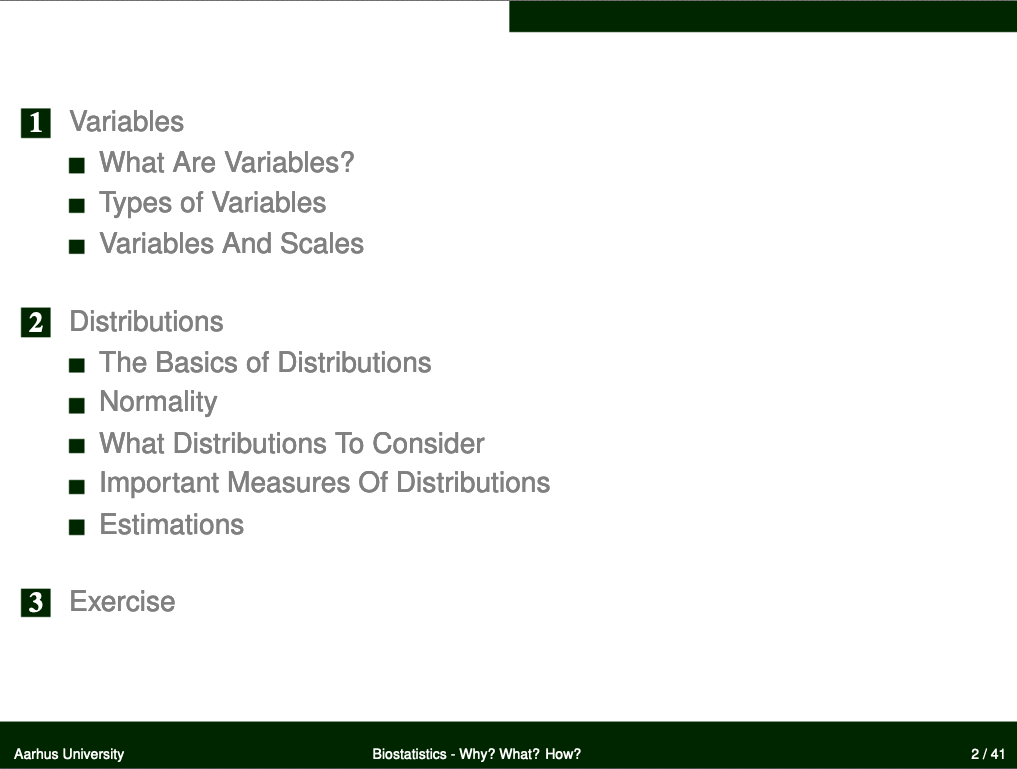## Data

Find the data for this exercise here.

## Loading the `R` Environment Object

``````load("Data/Primer.RData")  # load data file from Data folder
``````

## Variables

### Finding Variables

``````ls()  # list all elements in working environment
``````
``````##  "Colour"               "Depth"                "IndividualsPassingBy"
##  "Length"               "Reproducing"          "Sex"
##  "Size"                 "Temperature"
``````

### `Colour`

``````class(Colour)  # mode
``````
``````##  "character"
``````
``````barplot(table(Colour))  # fitting?
``````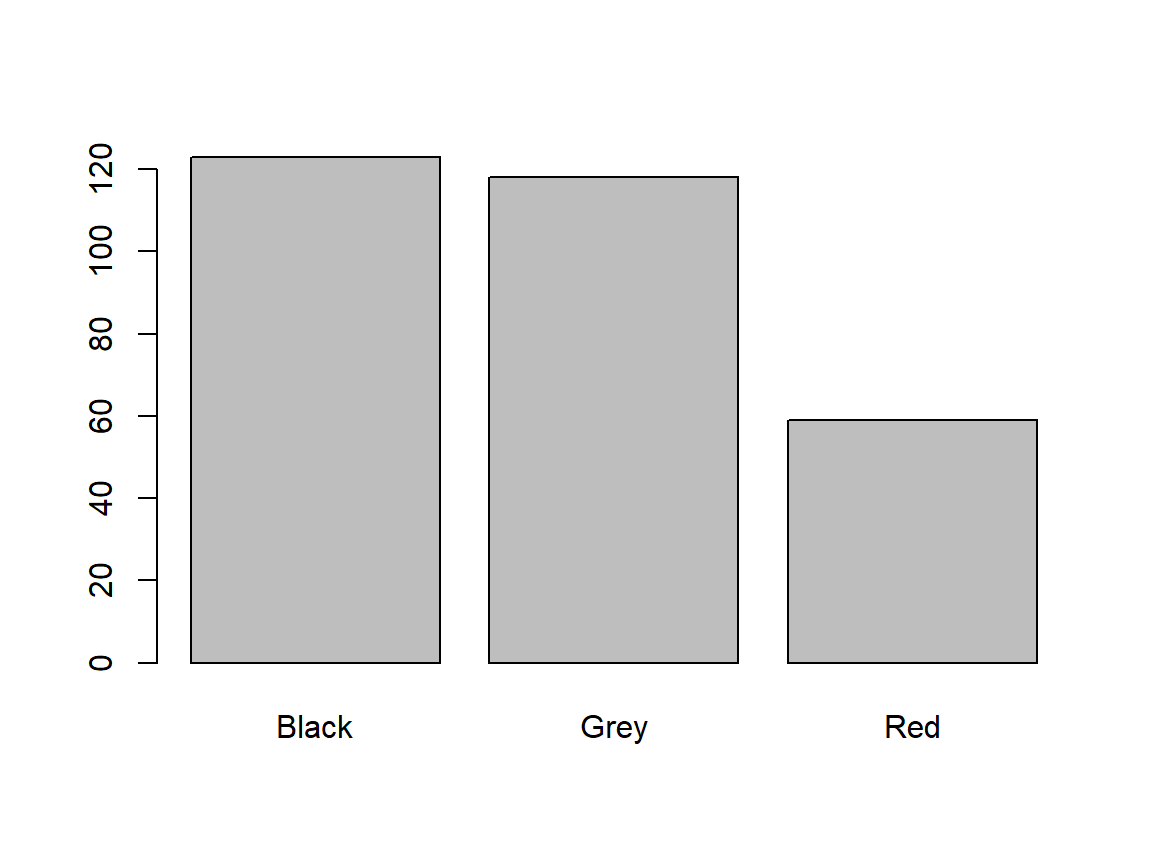Mode? character
Which scale? Nominal
What’s implied? Categorical data that can’t be ordered
Does data fit scale? Yes

### `Depth`

``````class(Depth)  # mode
``````
``````##  "numeric"
``````
``````barplot(Depth)  # fitting?
``````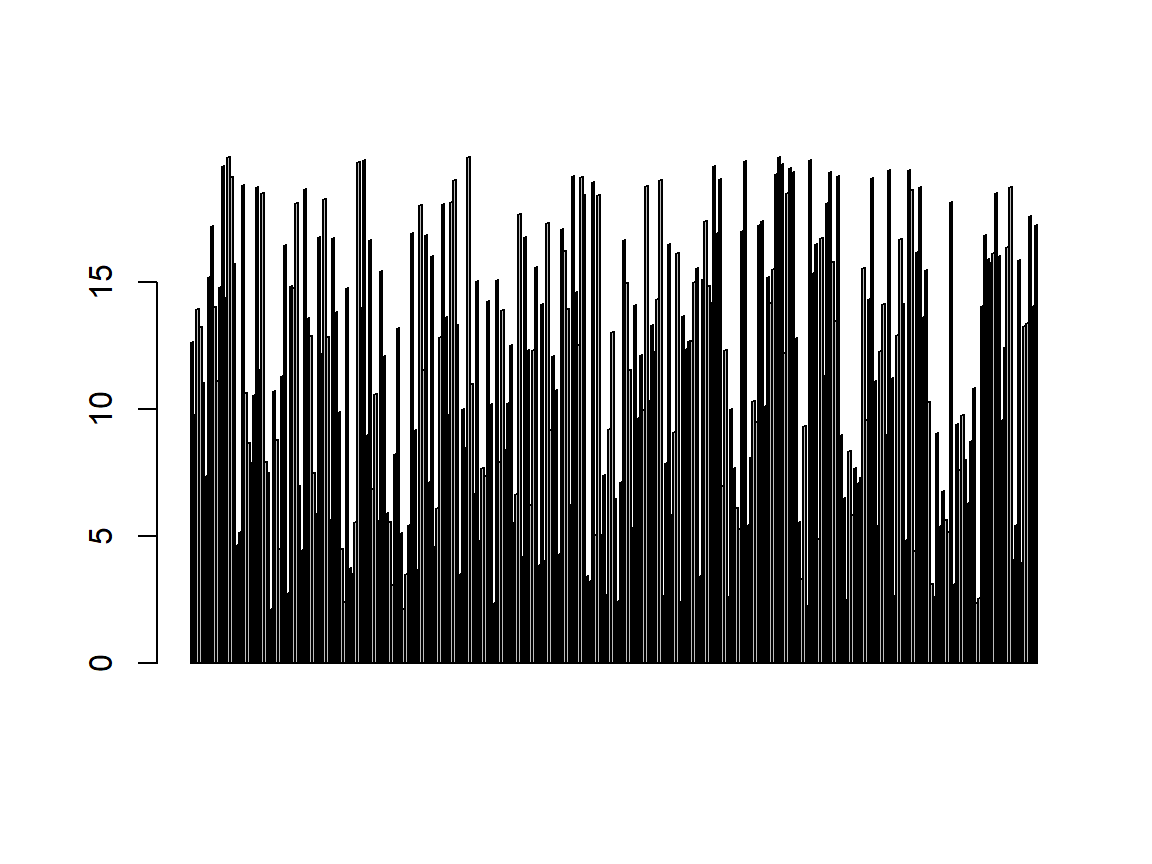Mode? numeric
Which scale? Interval/Discrete
What’s implied? Continuous data with a non-absence point of origin
Does data fit scale? Debatable (is 0 depth absence of depth?)

### `IndividualsPassingBy`

``````class(IndividualsPassingBy)  # mode
``````
``````##  "integer"
``````
``````barplot(IndividualsPassingBy)  # fitting?
``````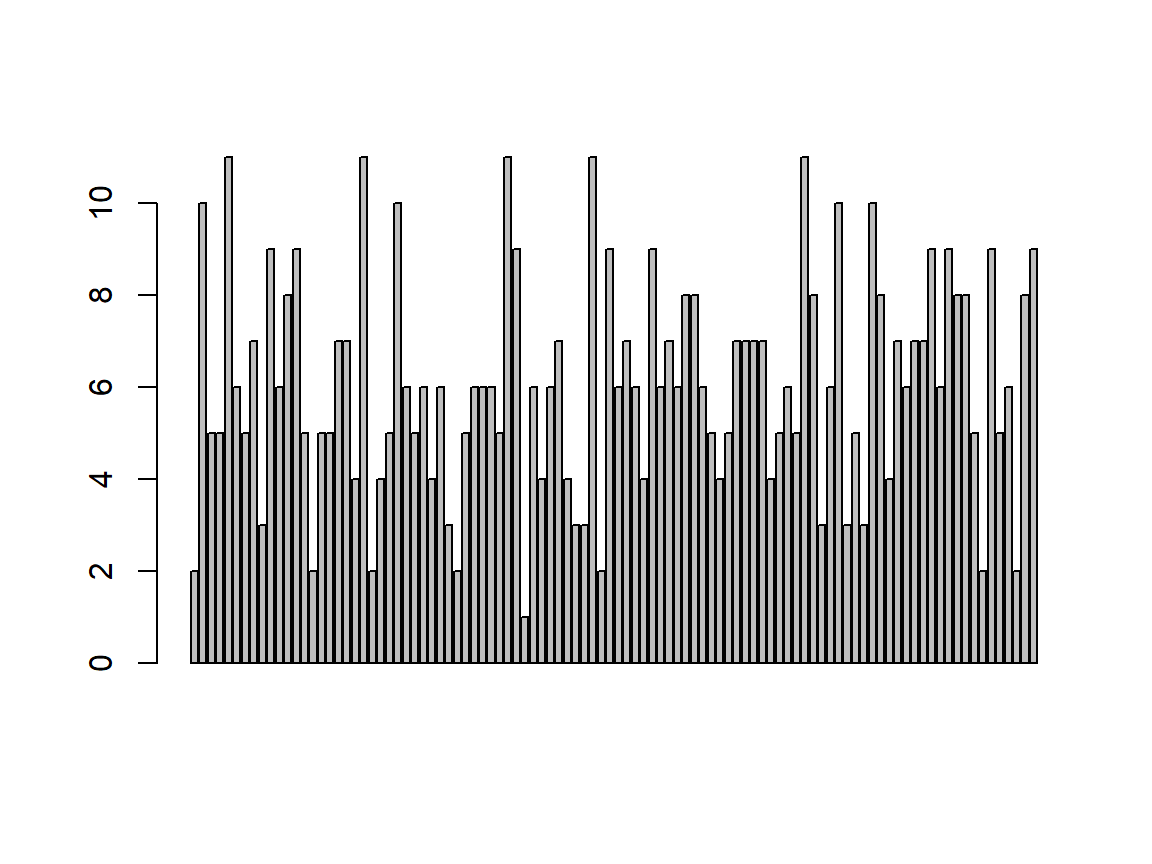Mode? integer
Which scale? Integer
What’s implied? Only integer numbers with an absence point of origin
Does data fit scale? Yes

### `Length`

``````class(Length)  # mode
``````
``````##  "numeric"
``````
``````barplot(Length)  # fitting?
``````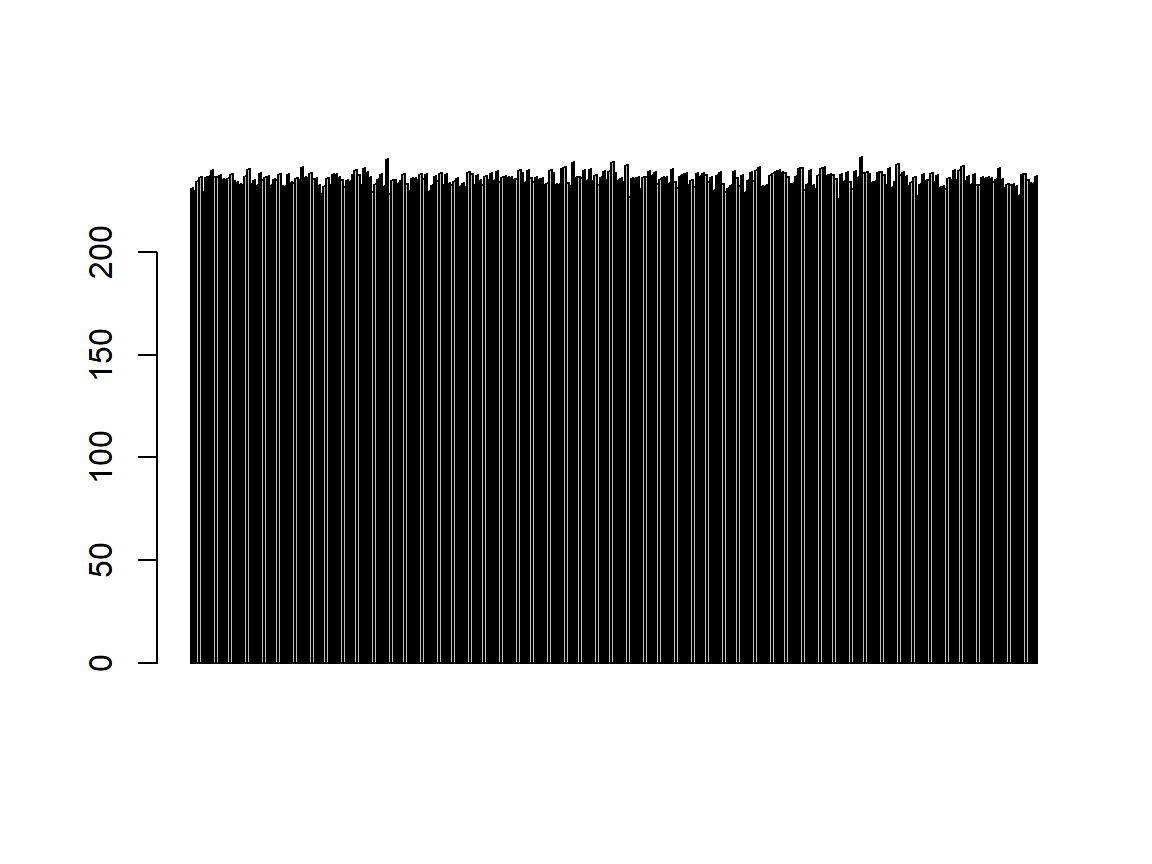Mode? numeric
Which scale? Relation/Ratio
What’s implied? Continuous data with an absence point of origin
Does data fit scale? Yes

### `Reproducing`

``````class(Reproducing)  # mode
``````
``````##  "integer"
``````
``````barplot(Reproducing)  # fitting?
``````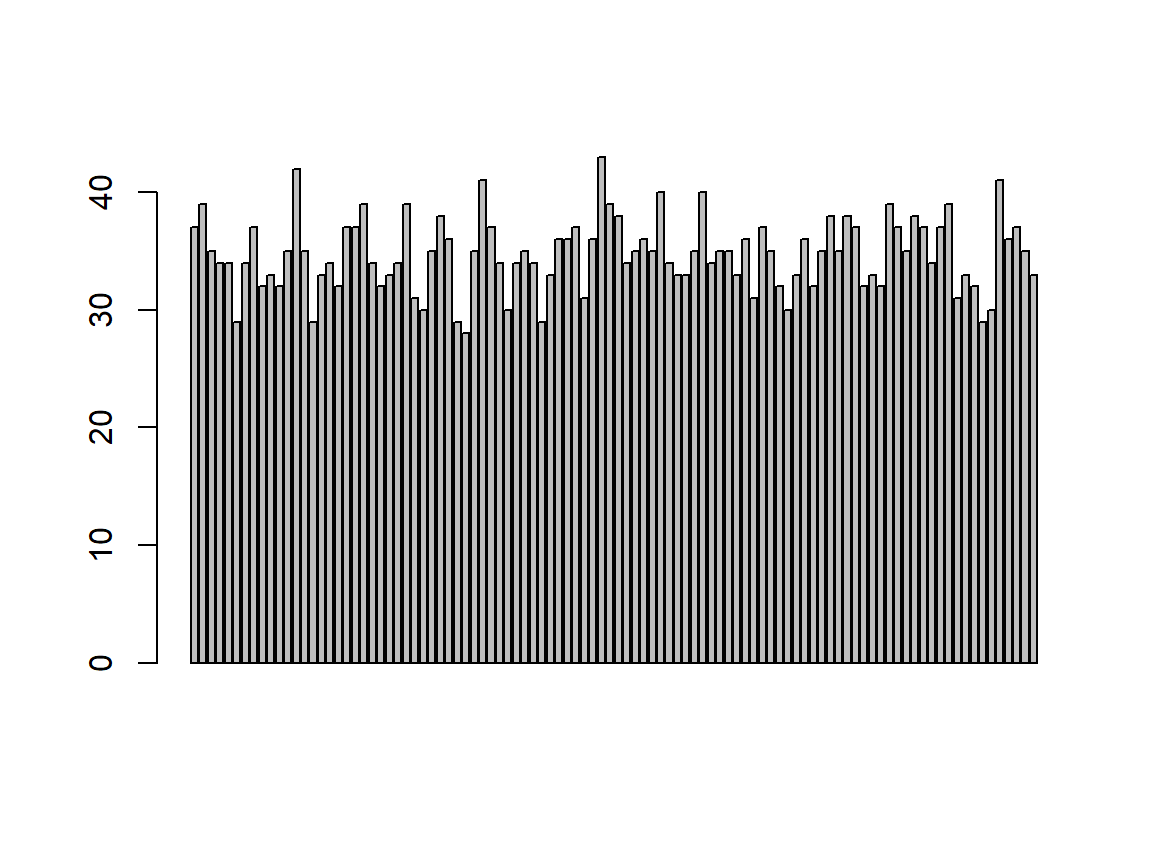Mode? integer
Which scale? Integer
What’s implied? Only integer numbers with an absence point of origin
Does data fit scale? Yes

### `Sex`

``````class(Sex)  # mode
``````
``````##  "factor"
``````
``````barplot(table(Sex))  # fitting?
``````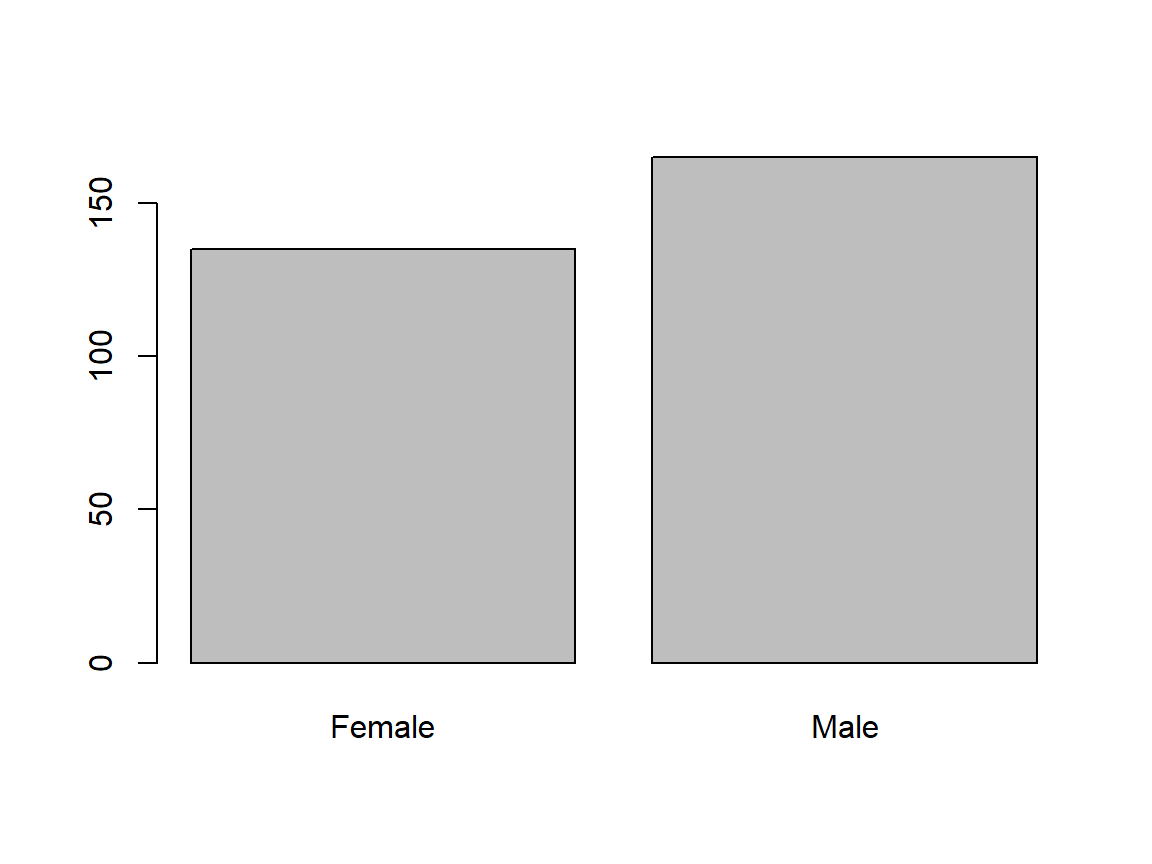Mode? factor
Which scale? Binary
What’s implied? Only two possible outcomes
Does data fit scale? Yes

### `Size`

``````class(Size)  # mode
``````
``````##  "character"
``````
``````barplot(table(Size))  # fitting?
``````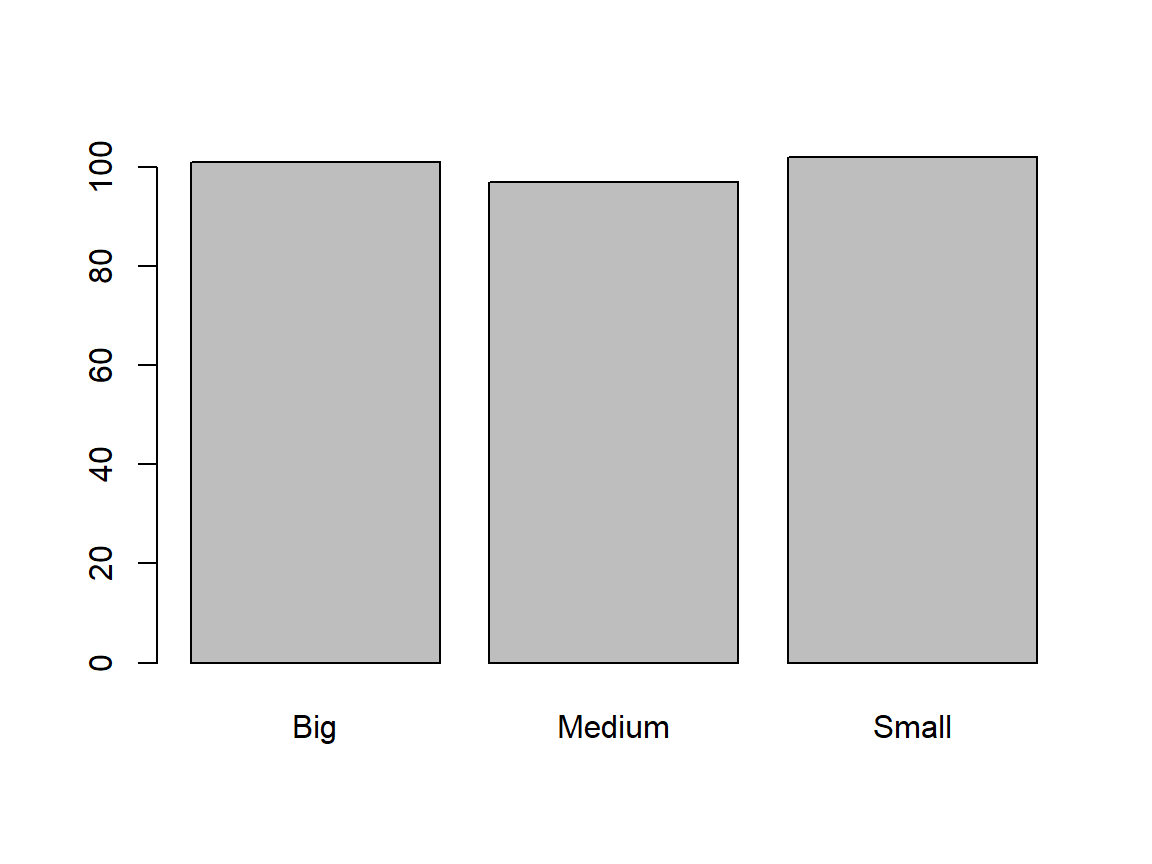Mode? character
Which scale? Ordinal
What’s implied? Categorical data that can be ordered
Does data fit scale? Yes

### `Temperature`

``````class(Temperature)  # mode
``````
``````##  "numeric"
``````
``````barplot(Temperature)  # fitting?
``````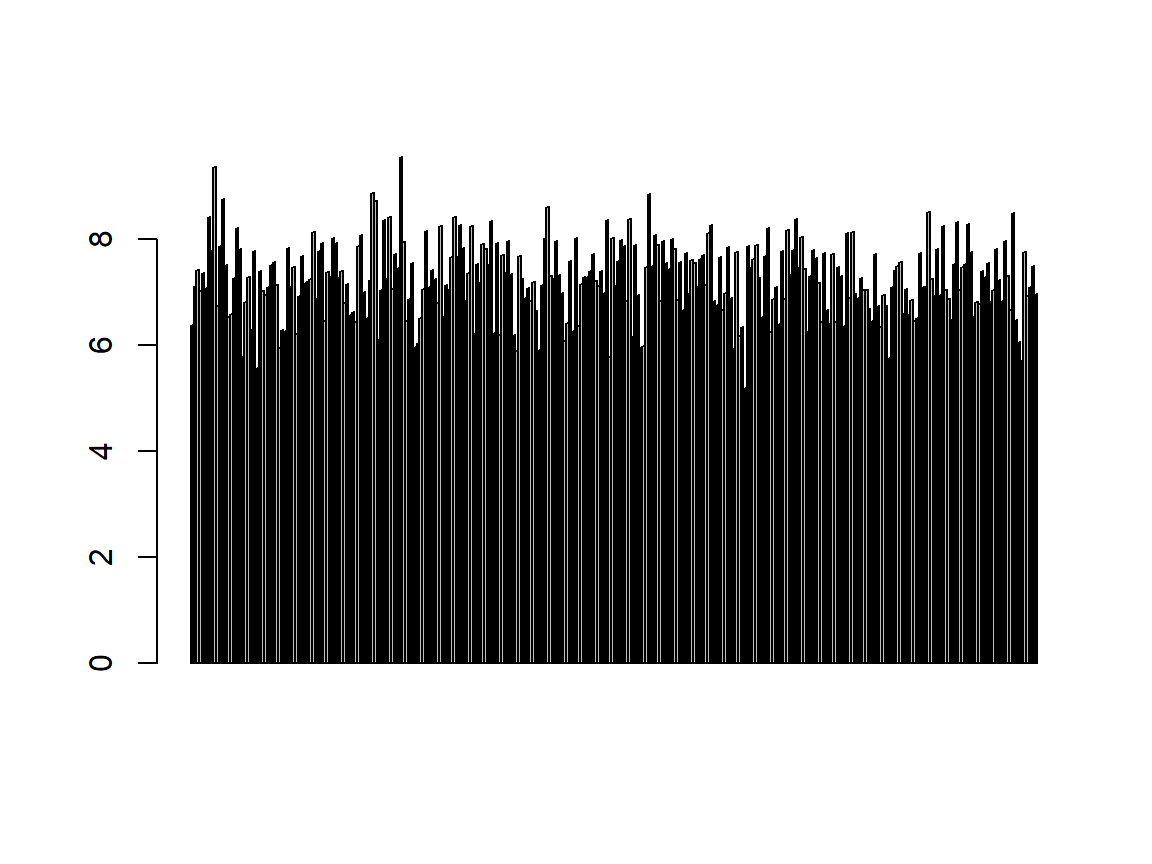Mode? numeric
Which scale? Interval/Discrete
What’s implied? Continuous data with a non-absence point of origin
Does data fit scale? Yes (the data is clearly recorded in degree Celsius)

## Distributions

### `Length`

``````plot(density(Length))  # distribution plot
``````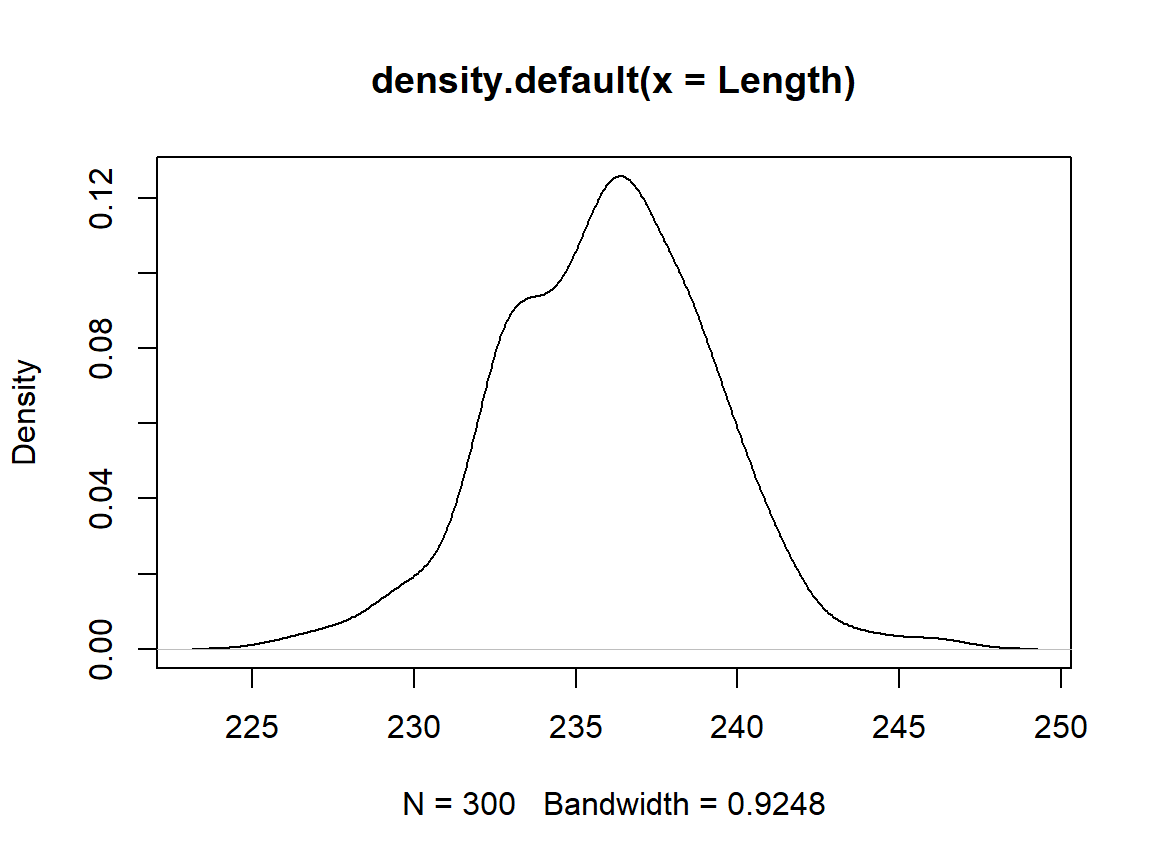``````shapiro.test(Length)  # normality check
``````
``````##
## 	Shapiro-Wilk normality test
##
## data:  Length
## W = 0.99496, p-value = 0.4331
``````

The data is normal distributed.

### `Reproducing`

``````plot(density(Reproducing))  # distribution
``````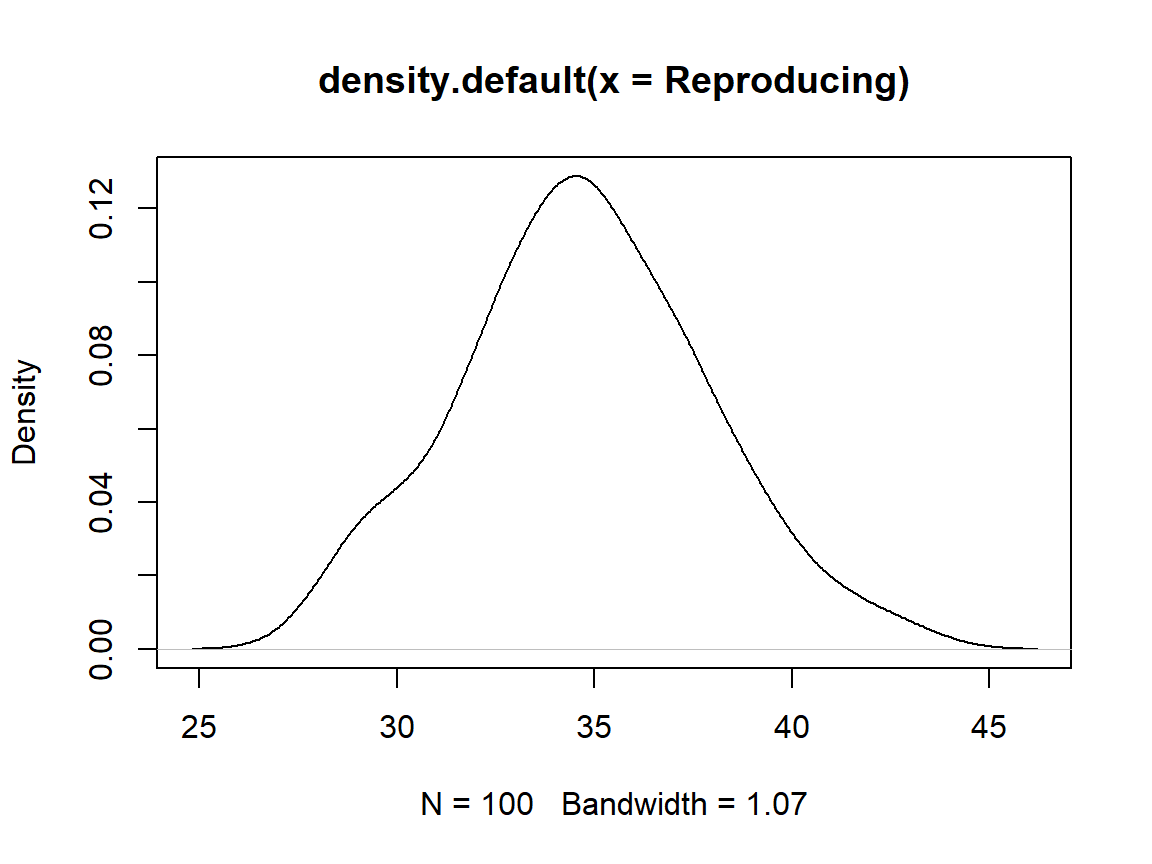``````shapiro.test(Reproducing)  # normality check
``````
``````##
## 	Shapiro-Wilk normality test
##
## data:  Reproducing
## W = 0.98444, p-value = 0.2889
``````

The data is binomial distributed (i.e. “How many individuals manage to reproduce”) but looks normal distributed. The normal distribution doesn’t make sense here because it implies continuity whilst the data only comes in integers.

### `IndividualsPassingBy`

``````plot(density(IndividualsPassingBy))  # distribution
``````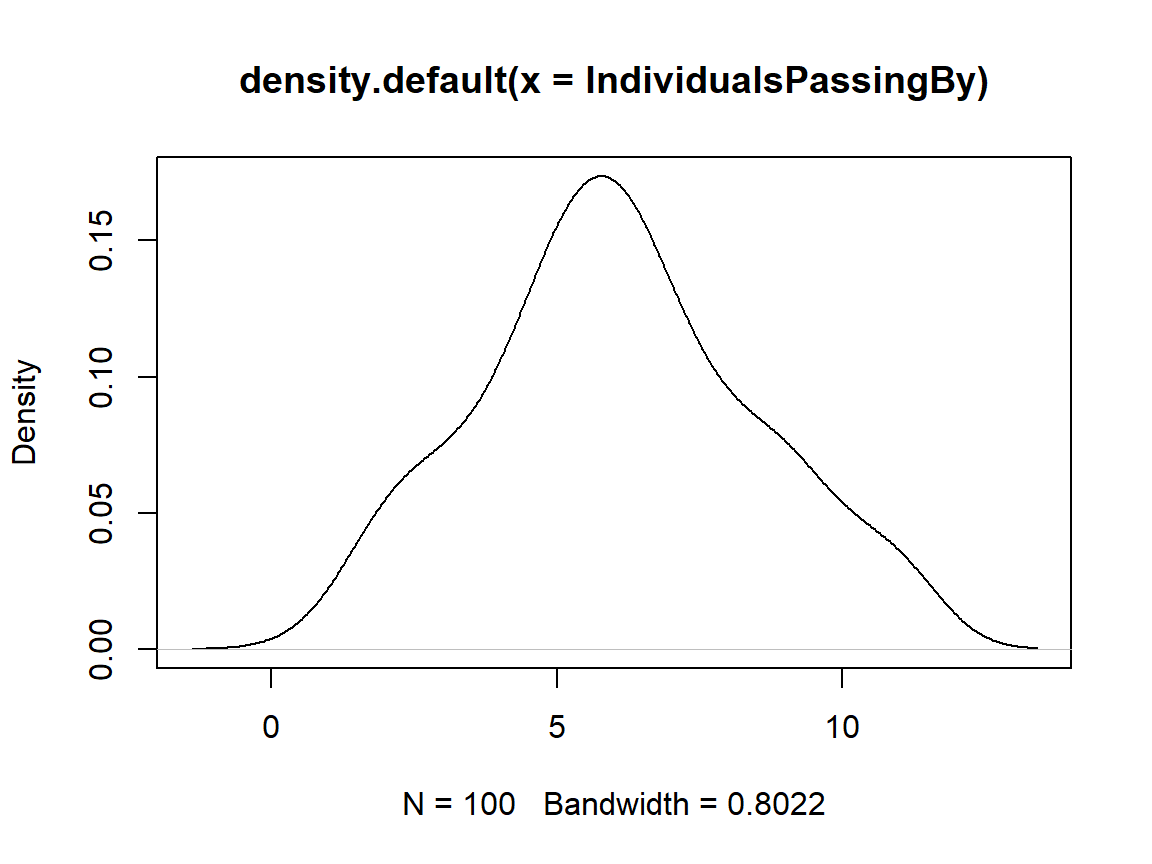``````shapiro.test(IndividualsPassingBy)  # normality check
``````
``````##
## 	Shapiro-Wilk normality test
##
## data:  IndividualsPassingBy
## W = 0.96905, p-value = 0.0187
``````

The data is poisson distributed (i.e. “How many individuals pass by an observer in a given time frame?").

### `Depth`

``````plot(density(Depth))  # distribution
``````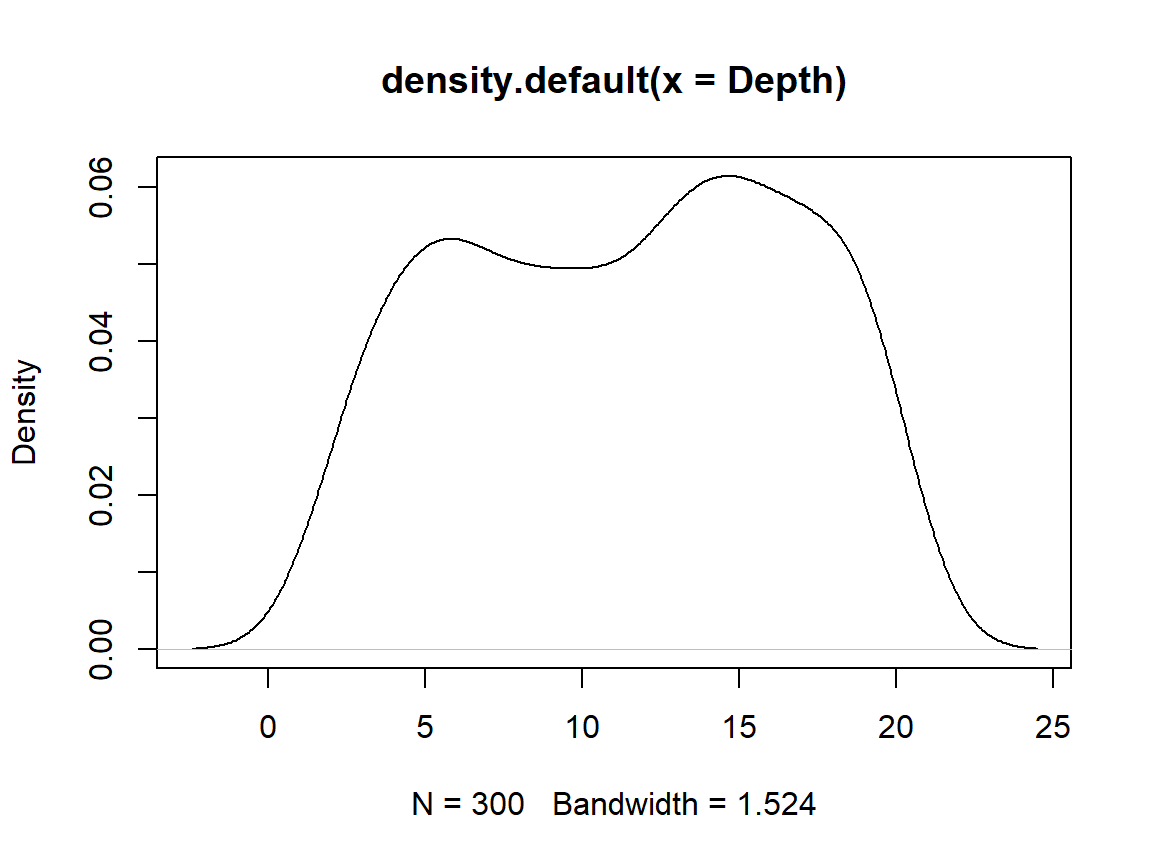The data is uniform distributed. You don’t know this distribution class from the lectures and I only wanted to confuse you with this to show you that there’s much more out there than I can show in our lectures.

Previous